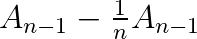Show Question
Incremental average (estimate update)

Statement:$\begin{displaymath} A_n = A_{n-1} + \frac{1}{n}(V_n - A_{n-1}) \end{displaymath}$

Alterative form:$\begin{displaymath}NewEstimate \leftarrow OldEstimate + StepSize[NewData - OldEstimate]\end{displaymath}$

The second form describes updating our estimate of the average by multiplying an error term, NewData - OldEstimate, by a weighting factor, StepSize. StepSize is$\frac{1}{n}$ when all data points are weighted equally.

The average,$A_{n-1}$ is known for a sequence of n-1 values,$V_1, V_2, ..., V_{n-1}$. If the next value,$V_n$ is included in the sequence, the new average,$A_{n}$, can be computed by adding the new component of the average,$\frac{1}{n}V_{n}$, to the old average transfered from an$n-1$ denominator to an$n$ denominator, ($A_{n-1} - \frac{1}{n}A_{n-1}$).

The ($A_{n-1} - \frac{1}{n}A_{n-1}$) component is easy to understand from an example: the difference between 1/4 and 1/5 is 1/(4*5). If we want to find the total divided by 5, and already have the total divided by 4, we can obtain the former by subtracting the known difference, 1/(4*5), which is the average divided by 4, divided by 5 again.